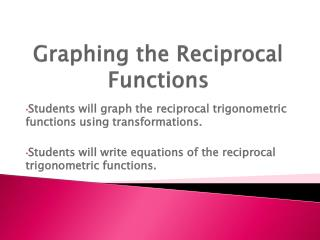DownloadDownload PresentationGraphing the Reciprocal Functions

# Graphing the Reciprocal Functions

Download Presentation## Graphing the Reciprocal Functions

- - - - - - - - - - - - - - - - - - - - - - - - - - - E N D - - - - - - - - - - - - - - - - - - - - - - - - - - -
##### Presentation Transcript

1. Graphing the Reciprocal Functions Students will graph the reciprocal trigonometric functions using transformations. Students will write equations of the reciprocal trigonometric functions.

2. HW Review • Reciprocal of cosine • Vertical Stretch by 2 • Vertical Shift down 3 • Horizontal Stretch by 4 • Horizontal Shift left π

3. GROUP WORK • Move to your assigned groups. (A, B, or C) • The assigned group leader will review the instructions for your group assignment. • Work cooperatively. Check to be sure that everyone in the group understands and completes the assignment. • Only ask for help if everyone in the group has been consulted and you ALL have the same question.

4. Goals: • Students will graph the reciprocal trigonometric functions using transformations. • Students will write equations of the reciprocal trigonometric functions.

5. y = cscx y = sec x y = cot x

6. How to mark your number lines Counting by

7. How to mark your number lines Counting by

8. Worksheet #2Matching Equations to Graphs • Hint: Do any of the equations show a horizontal shift? • Viewing Windows should be written as follows: • X axis: • Y axis:

9. Conclusion • HW: • Be sure that you have 4 problems from the graphing worksheet completed and checked. • Tuesday: Review • Continue work in groups to complete the matching exercise and writing equations from graphs. • Quiz: Wednesday 2/26